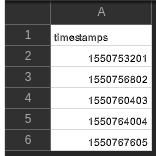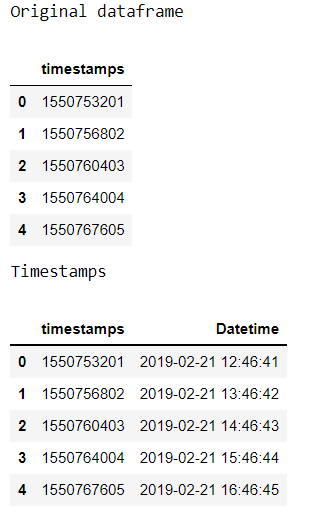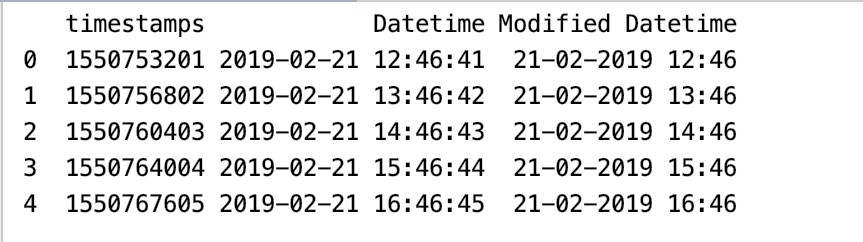# Using pandas to_datetime with timestamps

• Last Updated : 23 Aug, 2021

In this article, we are going to convert timestamps to datetime using the to_datetime() method from the pandas package. A timestamp is encoded information or a set of characters identifying when a certain event occurred, giving date and time of day, which can be accurate to a small fraction of a second.

Syntax:

Attention geek! Strengthen your foundations with the Python Programming Foundation Course and learn the basics.

To begin with, your interview preparations Enhance your Data Structures concepts with the Python DS Course. And to begin with your Machine Learning Journey, join the Machine Learning - Basic Level Course

pandas.to_datetime(arg, errors=’raise’, dayfirst=False, yearfirst=False, utc=None, box=True, format=None, exact=True, unit=None, infer_datetime_format=False, origin=’unix’, cache=False)

Parameters:

• arg: An integer, string, float, list or dict object to convert in to Date time object.
• dayfirst: Boolean value, places day first if True.
• yearfirst: Boolean value, places year first if True.
• utc: Boolean value, Returns time in UTC if True.
• format: String input to tell position of day, month and year.

Example 1: Timestamps with to_datetime.

Here we are converting the CSV file into a dataframe using pandas.DataFrame() method after reading the contents of the file using pandas.read_csv(), the timestamps column from the data Dataframe is given as an argument in the to_datetime() for it to be converted into DateTime. unit=’s’ is used to convert the values of the timestamp column to epoch time after converting the values to DateTime it is stored in a column called ‘Datetime’ in the Dataframe.

File Used:Code:

## Python3

 `# import packages``import` `pandas as pd`` ` `# creating a dataframe from the csv file``data ``=` `pd.DataFrame(pd.read_csv(``'timestamps.csv'``))`` ` `# viewing our dataframe``print``(``"Original dataframe"``)``display(data)`` ` `# unit='s' to convert it into epoch time``data[``'Datetime'``] ``=` `pd.to_datetime(data[``'timestamps'``], ``                                  ``unit``=``'s'``)`` ` `# checking our dataframe once again``print``(``"Timestamps"``)``display(data)`

Output:Example 2: Formatting the Datetime column.

The code is just the same as the previous example, the add-on is formating the ‘DateTime’ column. The Datetime column in the previous example can be further modified using strftime() which takes a string as an argument. strftime() returns an Index of formatted strings specified by date_format, which supports the same string format as the python standard library.

Syntax: strftime(format)

Code:

## Python3

 `# import packages``import` `pandas as pd``import` `datetime`` ` `# creating a dataframe from the csv file``data ``=` `pd.DataFrame(pd.read_csv(``'timestamps.csv'``))`` ` `# unit='s' to convert it into epoch time``data[``'Datetime'``] ``=` `pd.to_datetime(data[``'timestamps'``],``                                  ``unit``=``'s'``)`` ` `data[``'Modified Datetime'``] ``=` `data[``'Datetime'``].dt.strftime(``'%d-%m-%Y %H:%M'``)`` ` `# checking our dataframe once again``display(data)`

Output:Example 3: Using unit=’ms’  in the to_datetime() method.

The pd.to_datetime() method with a timestamp as argument and unit=’ms’, calculating the number of milliseconds to the Unix epoch start.

## Python3

 `# import packages``import` `pandas as pd`` ` `# unit='ms' to calculate the number ``# of milliseconds``date ``=` `pd.to_datetime(``1550767605``,``                      ``unit ``=` `'ms'``)`` ` `# checking our dataframe once again``print``(date)`

Output:

`1970-01-18 22:46:07.605000`

My Personal Notes arrow_drop_up